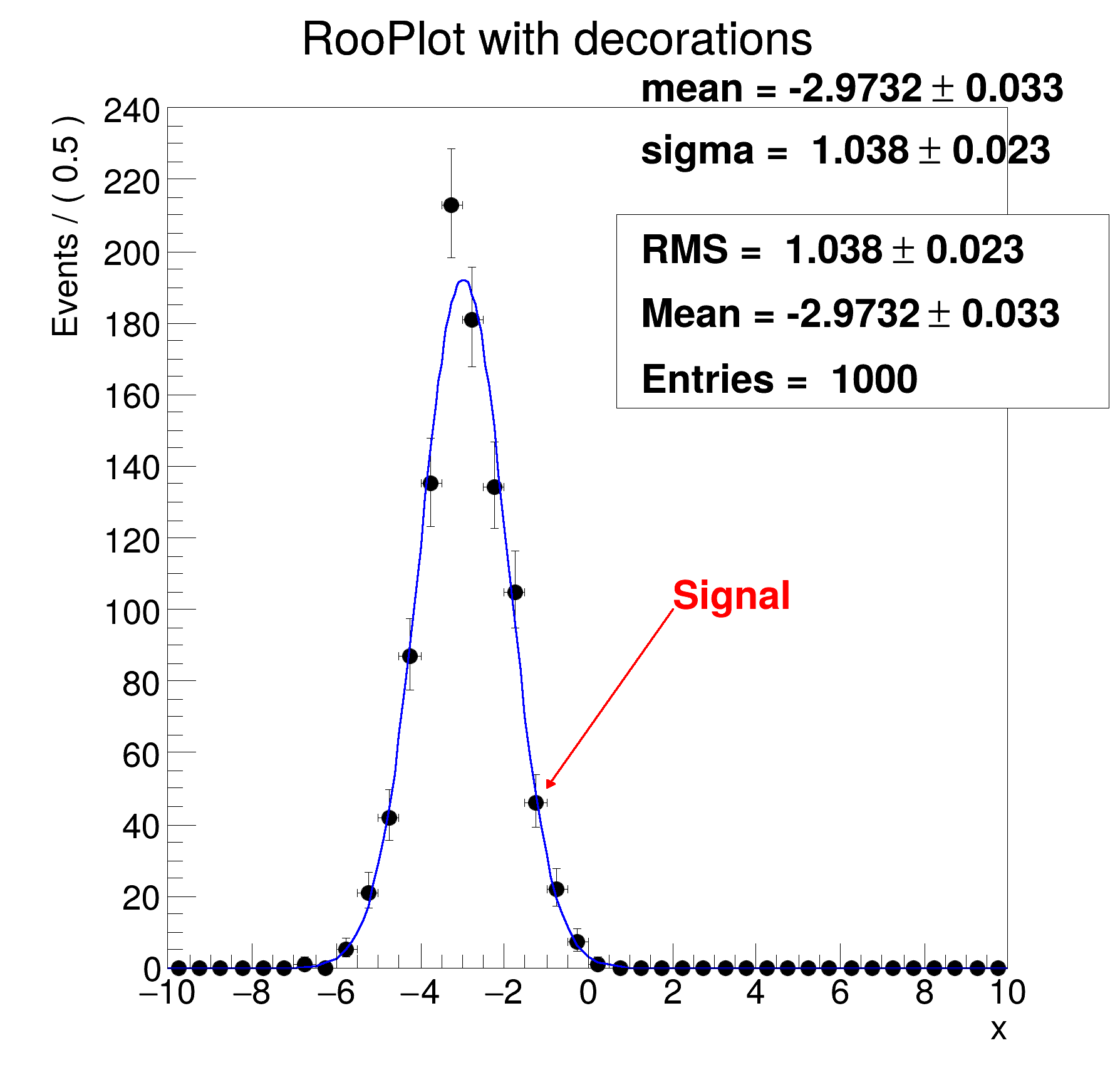ROOT   Reference Guide
Searching...
No Matches
rf106_plotdecoration.C File Reference

## Detailed DescriptionBasic functionality: adding boxes with parameters, statistics to RooPlots, decorating with arrows, text etc...#include "RooRealVar.h"
#include "RooDataSet.h"
#include "RooGaussian.h"
#include "TCanvas.h"
#include "TAxis.h"
#include "RooPlot.h"
#include "TText.h"
#include "TArrow.h"
#include "TFile.h"
using namespace RooFit;
{
// S e t u p m o d e l
// ---------------------
// Create observables
RooRealVar x("x", "x", -10, 10);
// Create Gaussian
RooRealVar sigma("sigma", "sigma", 1, 0.1, 10);
RooRealVar mean("mean", "mean", -3, -10, 10);
RooGaussian gauss("gauss", "gauss", x, mean, sigma);
// Generate a sample of 1000 events with sigma=3
std::unique_ptr<RooDataSet> data{gauss.generate(x, 1000)};
// Fit pdf to data
gauss.fitTo(*data, PrintLevel(-1));
// P l o t p . d . f a n d d a t a
// -------------------------------------
// Overlay projection of gauss on data
RooPlot *frame = x.frame(Name("xframe"), Title("RooPlot with decorations"), Bins(40));
data->plotOn(frame);
gauss.plotOn(frame);
// A d d b o x w i t h p d f p a r a m e t e r s
// -----------------------------------------------------
// Left edge of box starts at 55% of Xaxis)
gauss.paramOn(frame, Layout(0.55));
// A d d b o x w i t h d a t a s t a t i s t i c s
// -------------------------------------------------------
// X size of box is from 55% to 99% of Xaxis range, top of box is at 80% of Yaxis range)
data->statOn(frame, Layout(0.55, 0.99, 0.8));
// A d d t e x t a n d a r r o w
// -----------------------------------
TText *txt = new TText(2, 100, "Signal");
txt->SetTextSize(0.04);
TArrow *arrow = new TArrow(2, 100, -1, 50, 0.01, "|>");
arrow->SetLineColor(kRed);
arrow->SetFillColor(kRed);
arrow->SetLineWidth(3);
// P e r s i s t f r a m e w i t h a l l d e c o r a t i o n s i n R O O T f i l e
// ---------------------------------------------------------------------------------------------
TFile f("rf106_plotdecoration.root", "RECREATE");
frame->Write();
f.Close();
// To read back and plot frame with all decorations in clean root session do
// root> TFile f("rf106_plotdecoration.root") ;
// root> xframe->Draw() ;
new TCanvas("rf106_plotdecoration", "rf106_plotdecoration", 600, 600);
frame->GetYaxis()->SetTitleOffset(1.6);
frame->Draw();
}
#define f(i)
Definition RSha256.hxx:104
@ kRed
Definition Rtypes.h:66
Option_t Option_t TPoint TPoint const char GetTextMagnitude GetFillStyle GetLineColor GetLineWidth GetMarkerStyle GetTextAlign GetTextColor GetTextSize void data
Plain Gaussian p.d.f.
Definition RooGaussian.h:24
A RooPlot is a plot frame and a container for graphics objects within that frame.
Definition RooPlot.h:43
static RooPlot * frame(const RooAbsRealLValue &var, double xmin, double xmax, Int_t nBins)
Create a new frame for a given variable in x.
Definition RooPlot.cxx:239
void addObject(TObject *obj, Option_t *drawOptions="", bool invisible=false)
Add a generic object to this plot.
Definition RooPlot.cxx:383
TAxis * GetYaxis() const
Definition RooPlot.cxx:1279
void Draw(Option_t *options=nullptr) override
Draw this plot and all of the elements it contains.
Definition RooPlot.cxx:652
RooRealVar represents a variable that can be changed from the outside.
Definition RooRealVar.h:37
Draw all kinds of Arrows.
Definition TArrow.h:29
virtual void SetTitleOffset(Float_t offset=1)
Set distance between the axis and the axis title.
Definition TAttAxis.cxx:298
virtual void SetTextColor(Color_t tcolor=1)
Set the text color.
Definition TAttText.h:44
virtual void SetTextSize(Float_t tsize=1)
Set the text size.
Definition TAttText.h:47
The Canvas class.
Definition TCanvas.h:23
A ROOT file is composed of a header, followed by consecutive data records (TKey instances) with a wel...
Definition TFile.h:54
virtual Int_t Write(const char *name=nullptr, Int_t option=0, Int_t bufsize=0)
Write this object to the current directory.
Definition TObject.cxx:880
Base class for several text objects.
Definition TText.h:22
const Double_t sigma
Double_t x[n]
Definition legend1.C:17
The namespace RooFit contains mostly switches that change the behaviour of functions of PDFs (or othe...
[#1] INFO:Minimization -- RooAbsMinimizerFcn::setOptimizeConst: activating const optimization
[#1] INFO:Minimization -- RooAbsMinimizerFcn::setOptimizeConst: deactivating const optimization
Date
July 2008

Definition in file rf106_plotdecoration.C.